# Blocks & Cubes

Objective: Solve problems involving mass (weight), using g and kg.
Materials: Metric cubes, pattern blocks, simple balance.Knowing that each metric cube weighs 1 g, what is the weight of each pattern block? Caution! Weighing a single pattern block may not give you a very accurate measurement! How many different combinations of pattern blocks can you find where each set has a mass of 50 g? Check your answers on the balance. Can you use what you know to find sets of blocks which have a mass of 75 g? Describe any patterns you see between the masses of the blocks and sizes of the blocks.

Objective: Construct objects of a specific volume, expressed in cm3.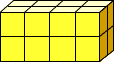Materials: Metric cubes, three 6-sided dice, simple balance.

 Roll all three dice at the same time. Build a three-dimensional solid (out of the linking metric cubes) that has as the measurements of its sides the values you rolled with the dice. Try building another 3-D solid but switching the values for the length, width and height. What do you notice? Take one of the figures above apart and using the same number of blocks construct any shape or figure of your choice. Compare the masses of the two objects using a balance. Knowing that all the cubes have the same mass and the same size, what is the volume of each of the objects you built?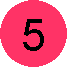Objective: Represent visually, a pattern to clarify relationships and to verify predictions.
Materials: Hexalink cubes, isometric dot paper.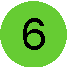There is only one way to arrange a single block: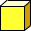There is only one way to arrange two blocks linked: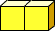There are only two ways to arrange three blocks linked: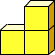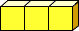How many different ways are there to arrange four blocks linked?